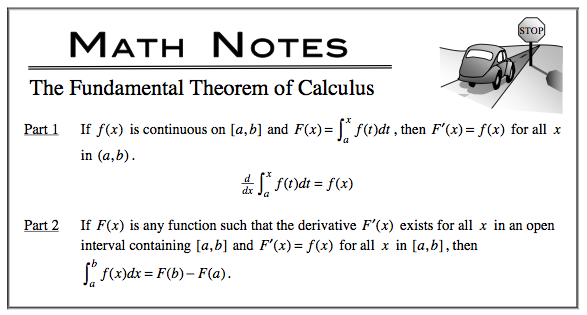### Home > CALC > Chapter 4 > Lesson 4.4.3 > Problem4-150

4-150.
1. Examine the following integrals. Consider the multiple tools available for evaluating integrals and use the best strategy for each. After evaluating the integral, write a short description of your method. Homework Help ✎

1.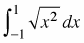2.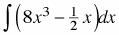3.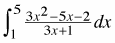4.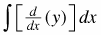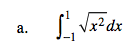$\sqrt{x^{2}}=\left | x \right |$

Simplify the integrand and then visualize the graph.
What type of symmetry does it have? What can you conclude about its area between x = −1 and x = 1?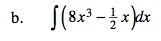This is an indefinite integral. Don't forget the +C.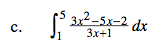Before you integrate, factor the numerator and simplify.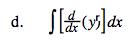Integrating a derivative gives you the original function + C.

yr + C